# 1. 前言¶

• 获取更好的特征表达，主要是将特征复用，特征细化做得更加极致，以HRNet，Res2Net等为代表作。

# 2. 介绍¶

ACNet的切入点为获取更好的特征表达，但和其它方法最大的区别在于它没有带来额外的超参数，而且在推理阶段没有增加计算量，这是十分具有吸引力的。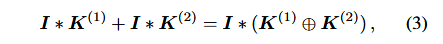# 3. ACNet原理¶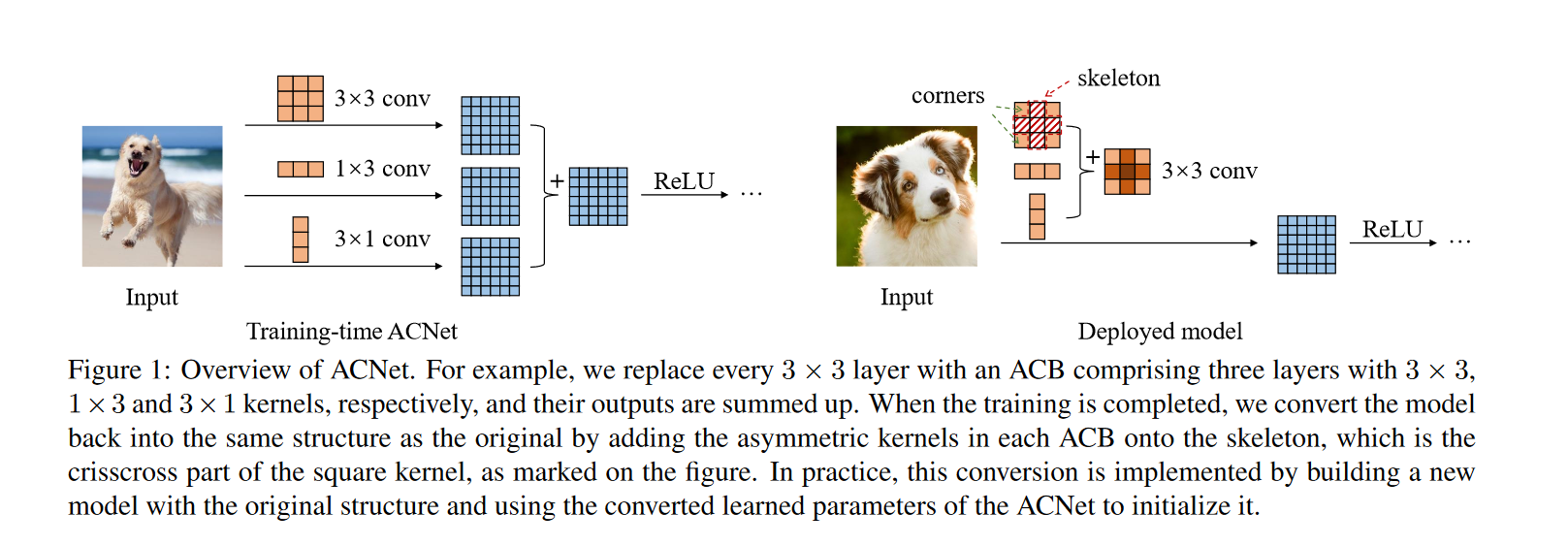• 训练阶段：因为$3\times 3$卷积是大多数网络的基础组件，因此ACNet的实验都是针对$3\times 3$卷积进行的。训练阶段就是将现有网络中的每一个$3\times 3$卷积换成$3\times 1$卷积+$1\times 3$卷积+$3\times 3$卷积共三个卷积层，最终将这三个卷积层的计算结果进行融合获得卷积层的输出。因为这个过程中引入的$1\times 3$卷积和$3\times 1$卷积是非对称的，所以将其命名为Asymmetric Convolution。
• 推理阶段：如Figure1右图所示，这部分主要是对三个卷积核进行融合。这部分在实现过程中就是使用融合后的卷积核参数来初始化现有的网络，因此在推理阶段，网络结构和原始网络是完全一样的了，只不过网络参数采用了特征提取能力更强的参数即融合后的卷积核参数，因此在推理阶段不会增加计算量。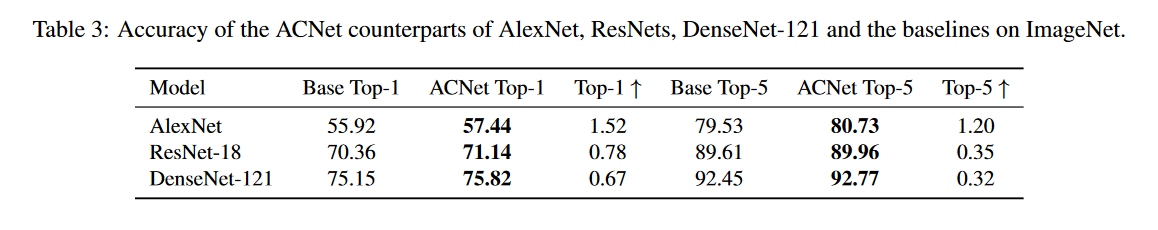# 4. 为什么ACNet能涨点？¶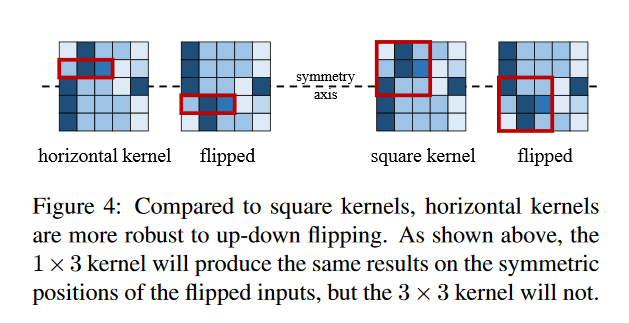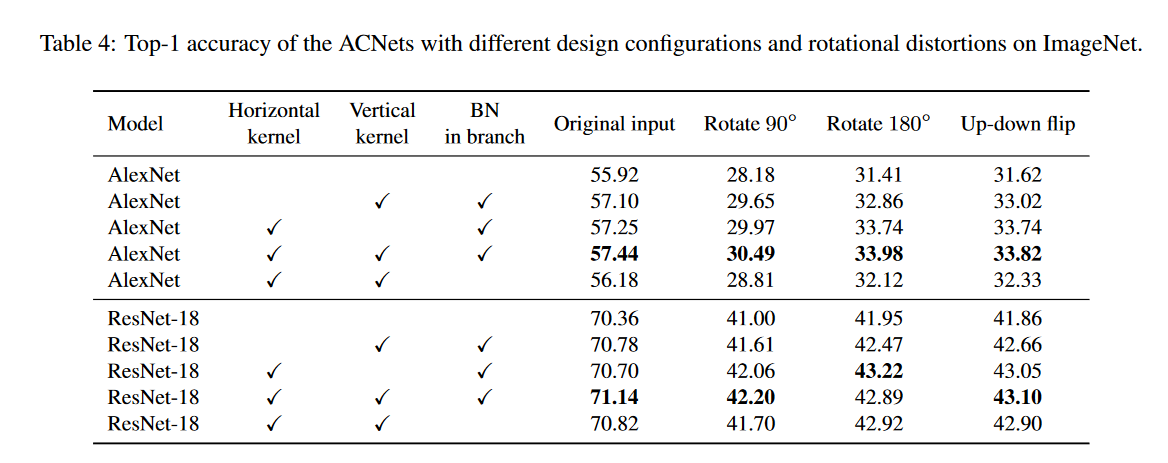# 5. 推理阶段的卷积核融合¶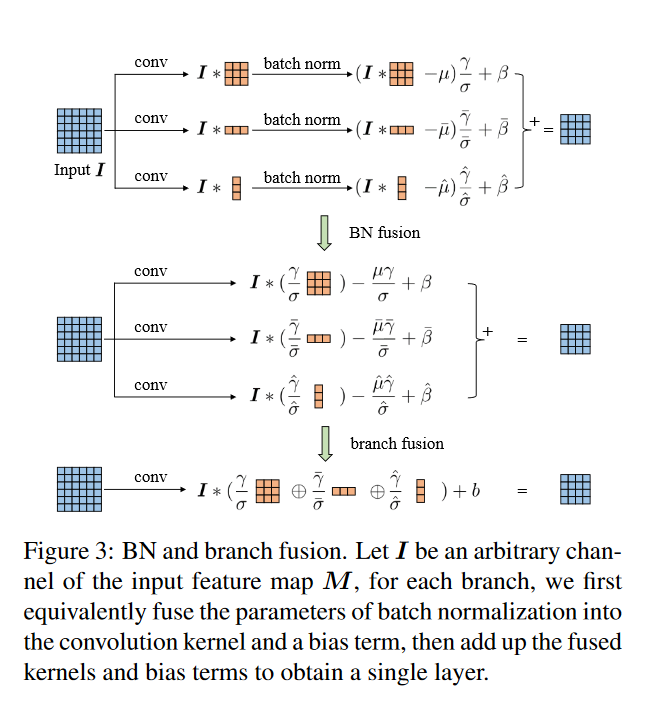# 6. Pytorch代码实现¶

# 去掉因为3x3卷积的padding多出来的行或者列
class CropLayer(nn.Module):

#   E.g., (-1, 0) means this layer should crop the first and last rows of the feature map. And (0, -1) crops the first and last columns
def __init__(self, crop_set):
super(CropLayer, self).__init__()
self.rows_to_crop = - crop_set
self.cols_to_crop = - crop_set
assert self.rows_to_crop >= 0
assert self.cols_to_crop >= 0

def forward(self, input):
return input[:, :, self.rows_to_crop:-self.rows_to_crop, self.cols_to_crop:-self.cols_to_crop]

# 论文提出的3x3+1x3+3x1
class ACBlock(nn.Module):

super(ACBlock, self).__init__()
self.deploy = deploy
if deploy:
self.fused_conv = nn.Conv2d(in_channels=in_channels, out_channels=out_channels, kernel_size=(kernel_size,kernel_size), stride=stride,
else:
self.square_conv = nn.Conv2d(in_channels=in_channels, out_channels=out_channels,
kernel_size=(kernel_size, kernel_size), stride=stride,
self.square_bn = nn.BatchNorm2d(num_features=out_channels)

center_offset_from_origin_border = padding - kernel_size // 2
ver_pad_or_crop = (center_offset_from_origin_border + 1, center_offset_from_origin_border)
hor_pad_or_crop = (center_offset_from_origin_border, center_offset_from_origin_border + 1)
if center_offset_from_origin_border >= 0:
self.ver_conv_crop_layer = nn.Identity()
self.hor_conv_crop_layer = nn.Identity()
else:
self.ver_conv = nn.Conv2d(in_channels=in_channels, out_channels=out_channels, kernel_size=(3, 1),
stride=stride,

self.hor_conv = nn.Conv2d(in_channels=in_channels, out_channels=out_channels, kernel_size=(1, 3),
stride=stride,
self.ver_bn = nn.BatchNorm2d(num_features=out_channels)
self.hor_bn = nn.BatchNorm2d(num_features=out_channels)

# forward函数
def forward(self, input):
if self.deploy:
return self.fused_conv(input)
else:
square_outputs = self.square_conv(input)
square_outputs = self.square_bn(square_outputs)
# print(square_outputs.size())
# return square_outputs
vertical_outputs = self.ver_conv_crop_layer(input)
vertical_outputs = self.ver_conv(vertical_outputs)
vertical_outputs = self.ver_bn(vertical_outputs)
# print(vertical_outputs.size())
horizontal_outputs = self.hor_conv_crop_layer(input)
horizontal_outputs = self.hor_conv(horizontal_outputs)
horizontal_outputs = self.hor_bn(horizontal_outputs)
# print(horizontal_outputs.size())
return square_outputs + vertical_outputs + horizontal_outputs


# 8. 总结¶